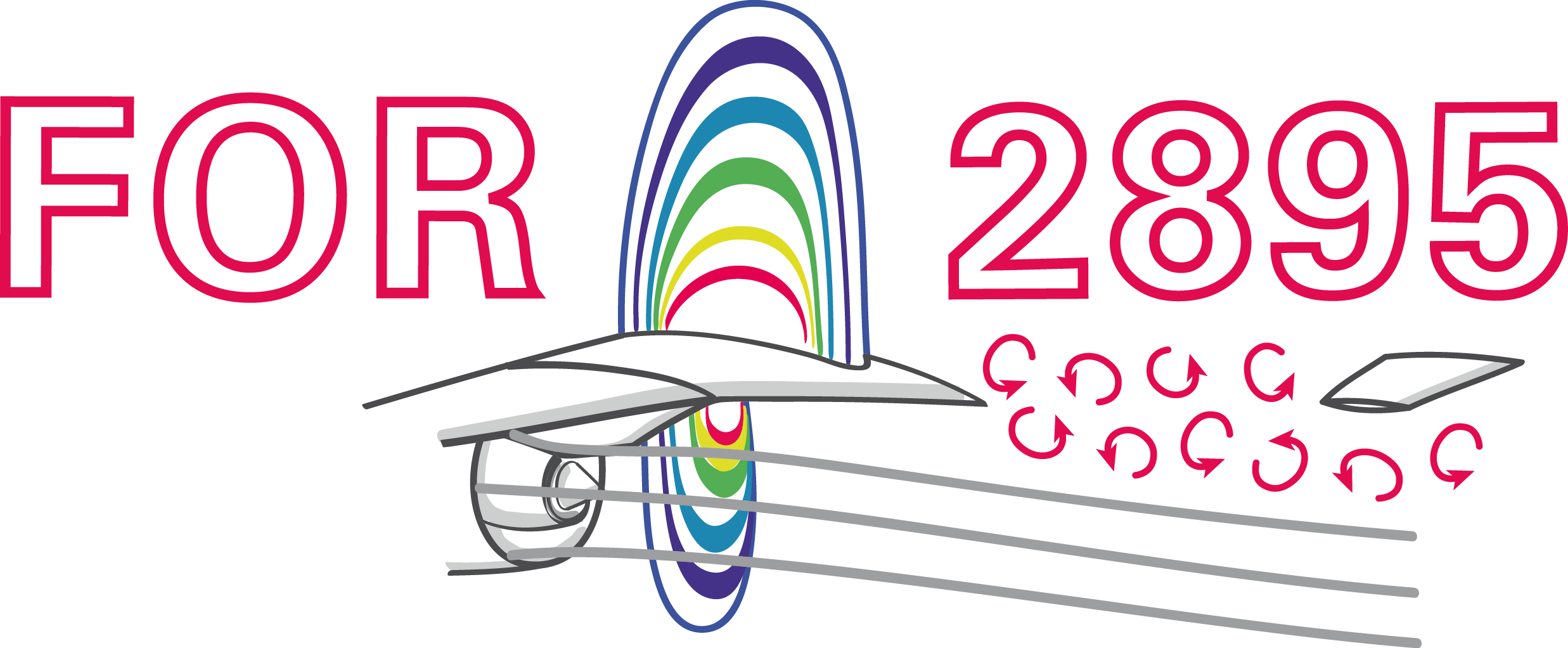### Data-driven modeling and validation of reactive mass transfer at rising bubbles

Andre Weiner, TU Braunschweig, Institute of Fluid MechanicsThese slides and most of the linked resources are licensed under a

## Outline

1. Data-driven modeling overview
2. Modeling of reactive mass transfer
3. Outlook
• Extracting coherent flow structures
• Closure modeling as a control problem

## Combining ML and CFD why and how

### Why combine CFD and ML?

CFD

• produces large amounts of complex data
• requires data or representations thereof

ML

• finds patterns in data
• creates useful representations of data

### What is data?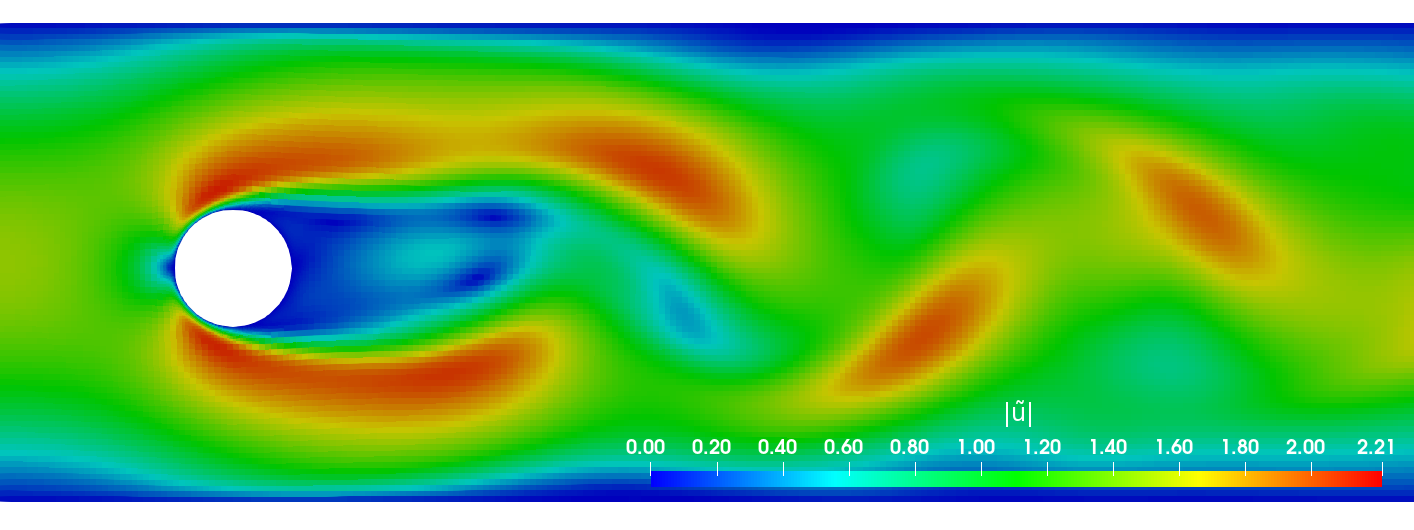primary data: scalar/vector fields, boundary fields, integral values


# log.rhoPimpleFoam
Courant Number mean: 0.020065182 max: 0.77497916
deltaT = 6.4813615e-07
Time = 1.22219e-06

PIMPLE: iteration 1
diagonal:  Solving for rho, Initial residual = 0, Final residual = 0, No Iterations 0
DILUPBiCGStab:  Solving for Ux, Initial residual = 0.0034181127, Final residual = 6.0056507e-05, No Iterations 1
DILUPBiCGStab:  Solving for Uy, Initial residual = 0.0052004883, Final residual = 0.00012352706, No Iterations 1
DILUPBiCGStab:  Solving for e, Initial residual = 0.06200185, Final residual = 0.0014223046, No Iterations 1
limitTemperature limitT Lower limited 0 (0%) of cells
limitTemperature limitT Upper limited 0 (0%) of cells
limitTemperature limitT Unlimited Tmax 329.54945
Unlimited Tmin 280.90821


Checking geometry...
...
Mesh has 2 solution (non-empty) directions (1 1 0)
All edges aligned with or perpendicular to non-empty directions.
Boundary openness (1.4469362e-19 3.3639901e-21 -2.058499e-13) OK.
Max cell openness = 2.4668495e-16 OK.
Max aspect ratio = 3.0216602 OK.
Minimum face area = 7.0705331e-08. Maximum face area = 0.00033983685.  Face area magnitudes OK.
Min volume = 1.2975842e-10. Max volume = 6.2366859e-07.  Total volume = 0.0017254212.  Cell volumes OK.
Mesh non-orthogonality Max: 60.489216 average: 4.0292071
Non-orthogonality check OK.
Face pyramids OK.
Max skewness = 1.1453509 OK.
Coupled point location match (average 0) OK.



secondary data: log files, input dictionaries, mesh quality metrics, ...

### Examples for data-driven workflowsExample: creating a surrogate or reduced-order model based on numerical data.Example: creating a space and time dependent boundary condition based on numerical or experimental data.Example: creating closure models based on numerical data.Example: active flow control or shape optimization.

### Supervised learningCreating a mapping from features to labels based on examples.

### Unsupervised learningFinding patterns in unlabeled data.

### (Deep) Reinforcement learningCreate an intelligent agent that learns to map states to actions such that cumulative rewards are maximized.

### What if my problem does not fit into these categories?

$\rightarrow$ mathematical, physical, numerical modeling

## Reactive mass transfer at rising bubbles

A single-phase simulation approach to compute the mass transfer at rising bubbles

Joint work with D. Bothe, CMY. Claassen, IR. Hierck, JAM. Kuipers, MW. Baltussen.

### Gas-liquid reactors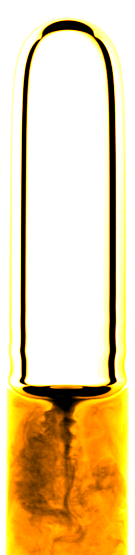micro reactor
size: millimeter
source: SPP 1740

prediction of

• mass transfer
• enhancement
• mixing
• conversion
• selectivity
• yield
• ...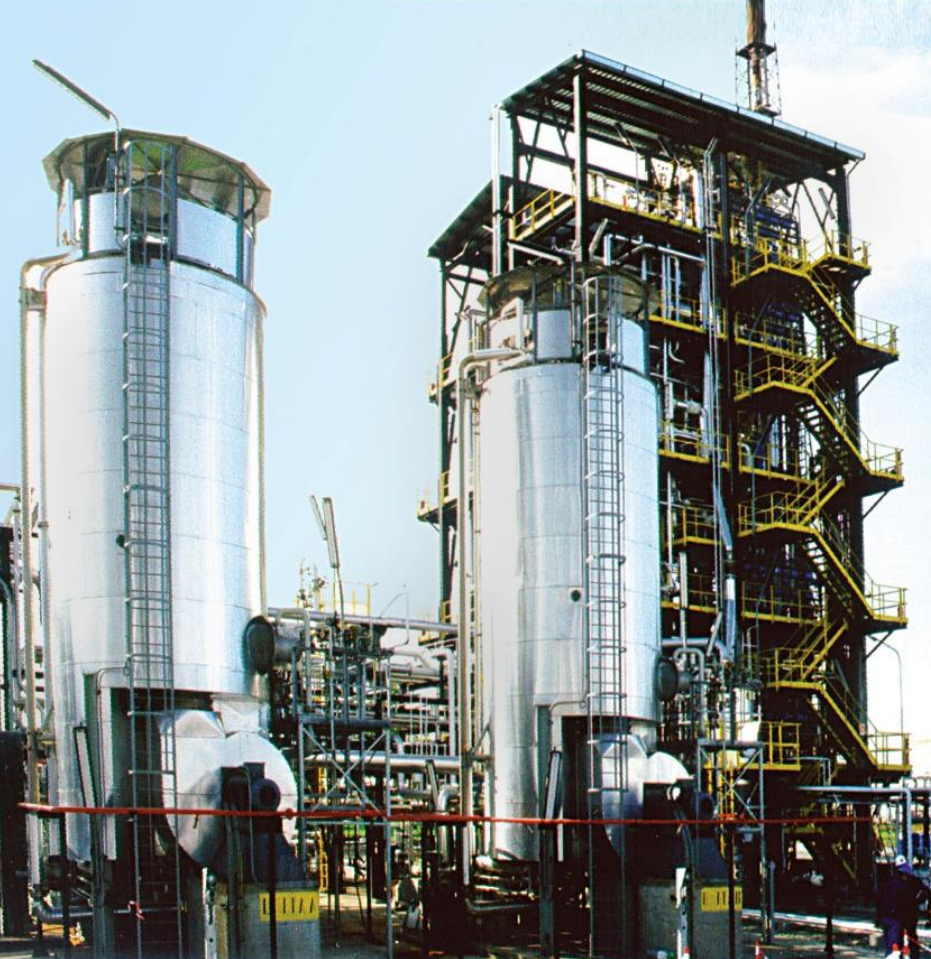bubble column reactor
size: meter
source: R. M. Raimundo, ENI

### Specimen calculation

$d_b=1~mm$ water/oxygen at room temperature

• $Pe = Sc\ Re = \nu_l / D_{O_2} \cdot U_b d_b/\nu_l \approx 10^5$
• $$Re\approx 250;\quad \delta_h/d_b \propto Re^{-1/2};\quad\delta_h\approx 45~\mu m$$
• $$Sc\approx 500;\quad \delta_c/\delta_h \propto Sc^{-1/2};\quad\delta_c\approx 2.5~\mu m$$

$\delta_h/\delta_c$ typically 10 ... 100

feasible simulations up to $Pe\approx 10000$ (3D, HPC)

Why we might be interested in a simplified simulation approach:

• perform parameter studies
• validate boundary layer models
• generate data for ML-based models
• ...

### Idea: decoupling of two-phase flow and species transport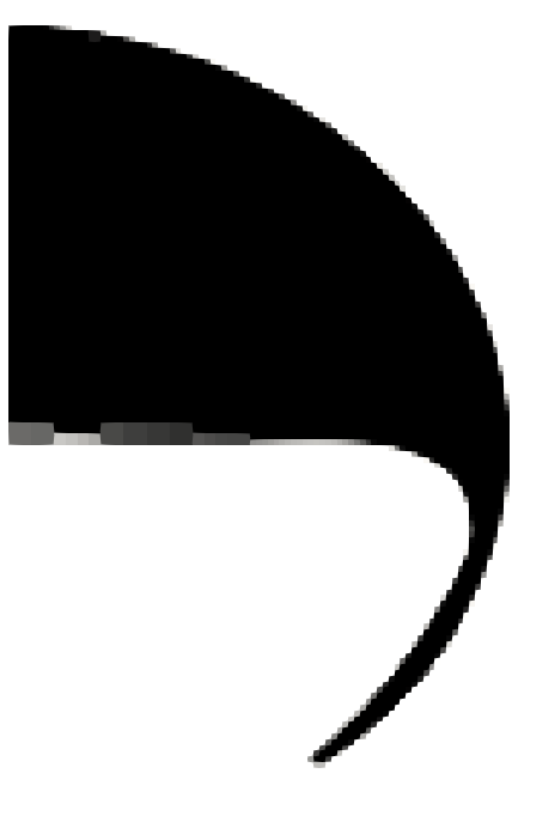1. Two-phase flow simulation (Volume-of-Fluid)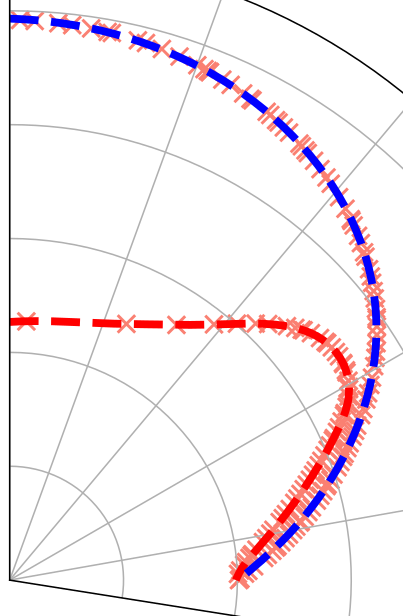2. Parametrization of shape and interfacial velocity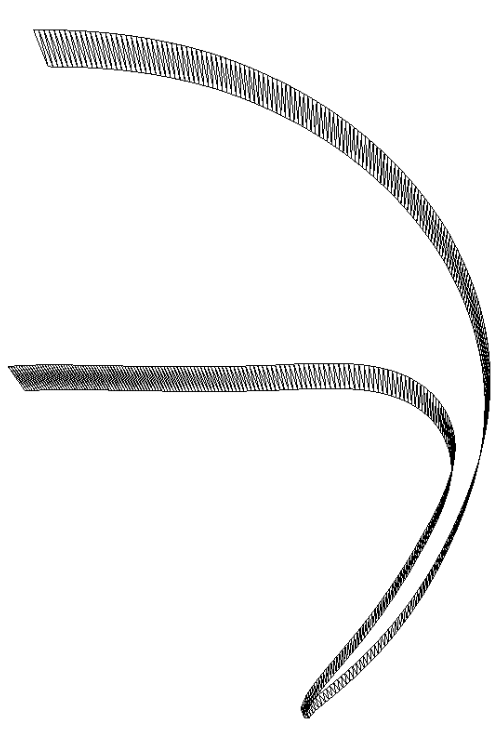3. Geometry generation and export (STL format)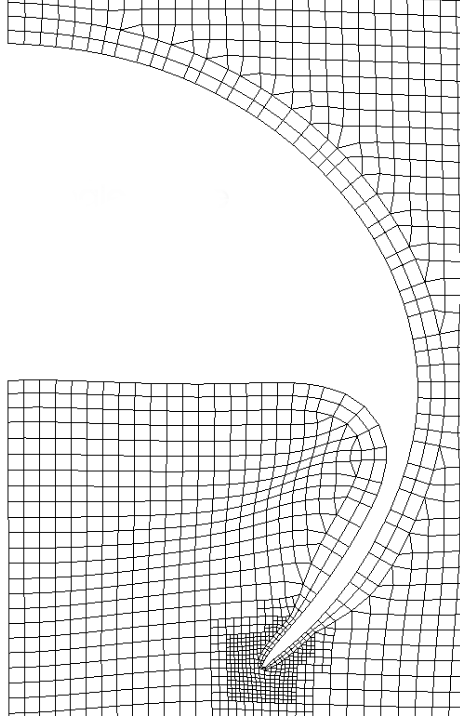4. Single phase mesh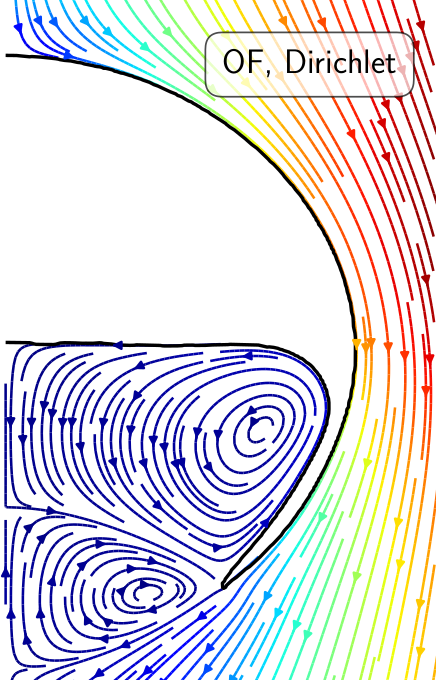5. Flow solution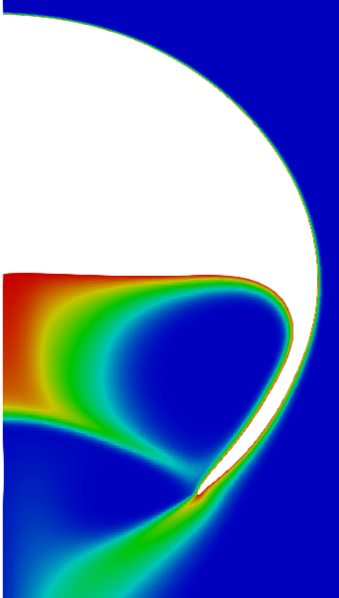6. Species transport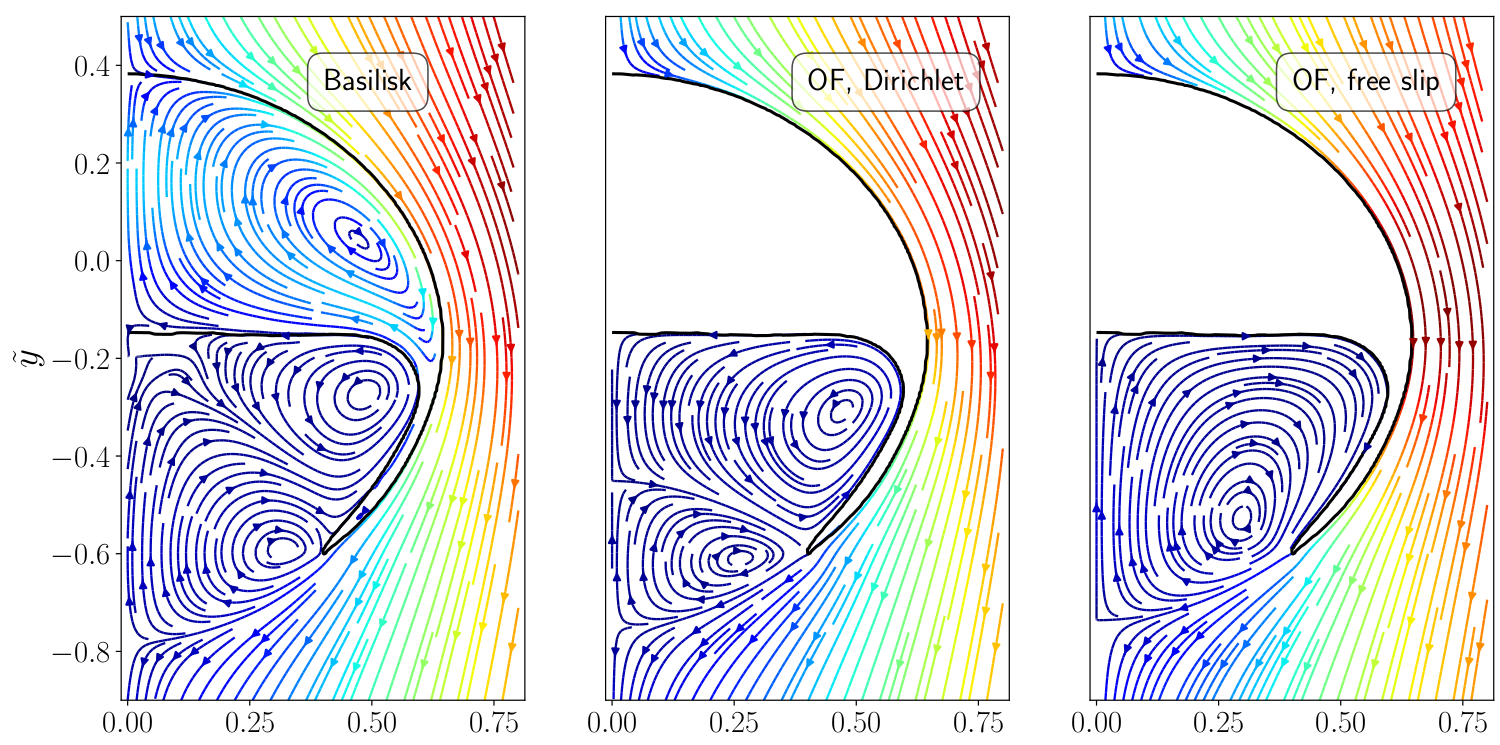Comparison of two-phase and single-phase flow fields.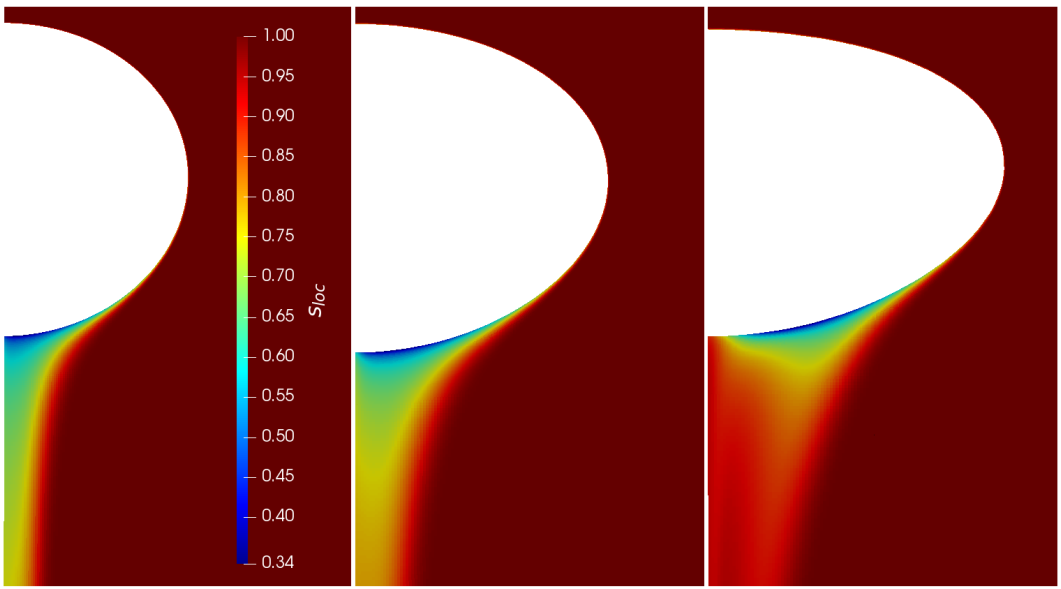Influence of bubble size on local selectivity.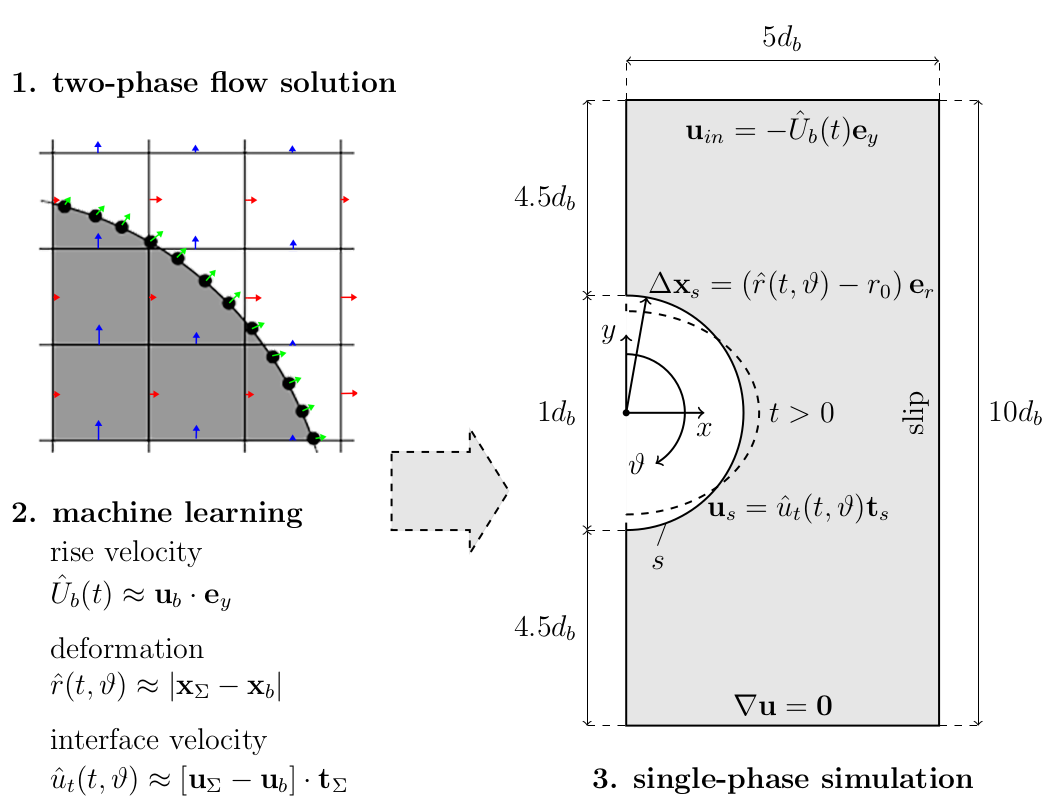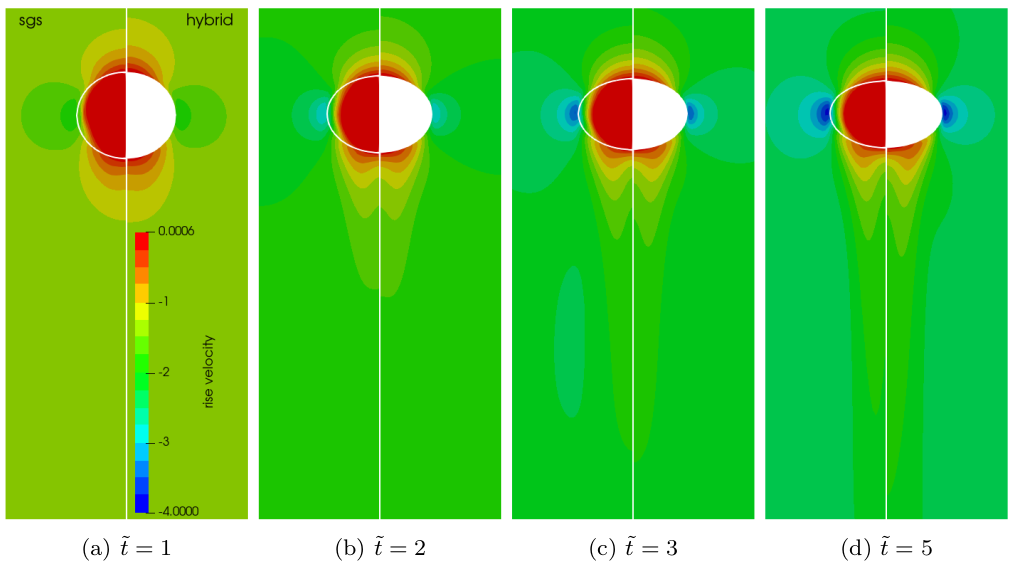Two-phase velocity field (left half) versus single-phase velocity field (right half); speed-up of 20-40x with 120x finer mesh at surface.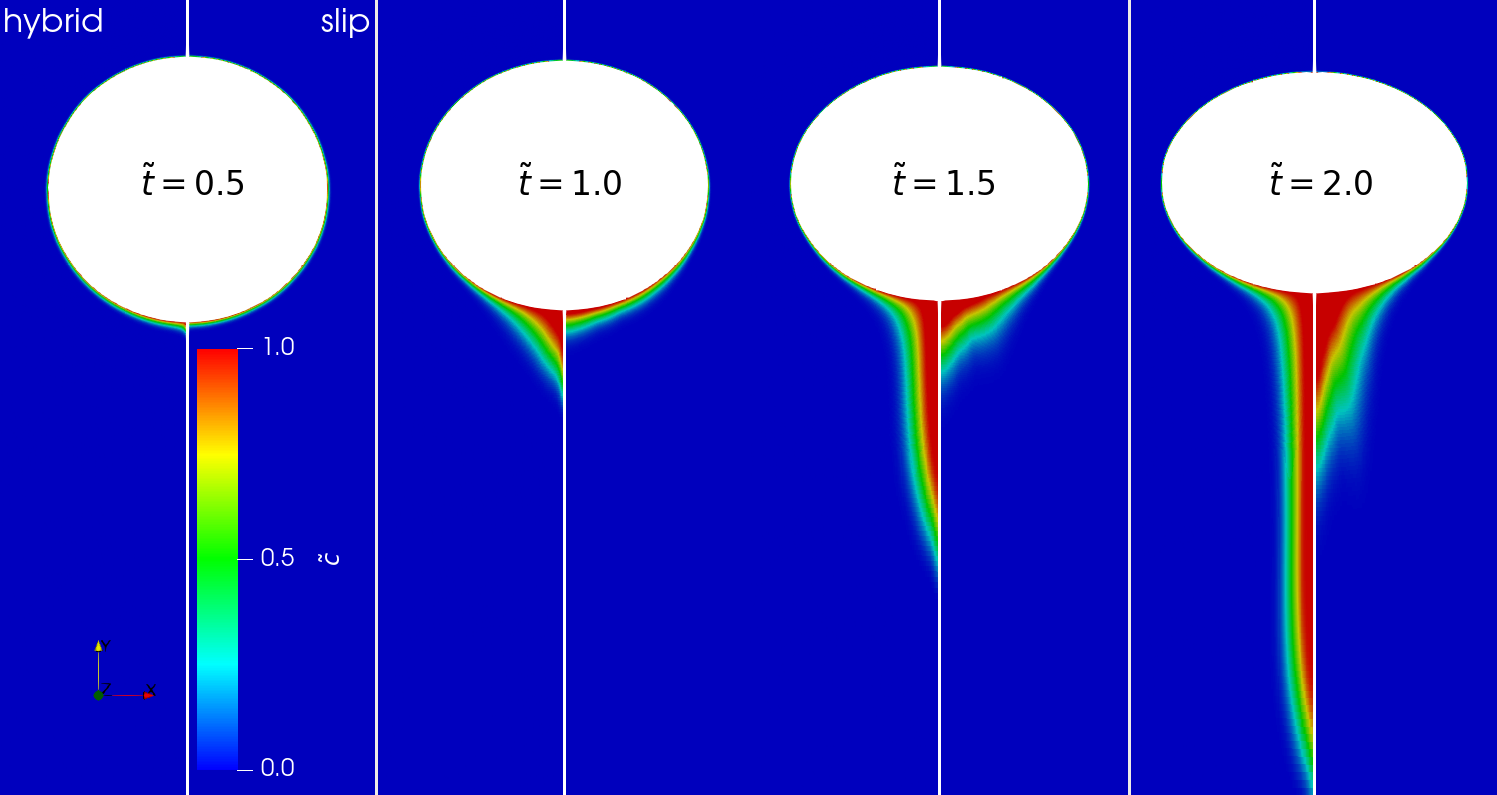Comparison of concentration fields: data-driven vs. free-slip.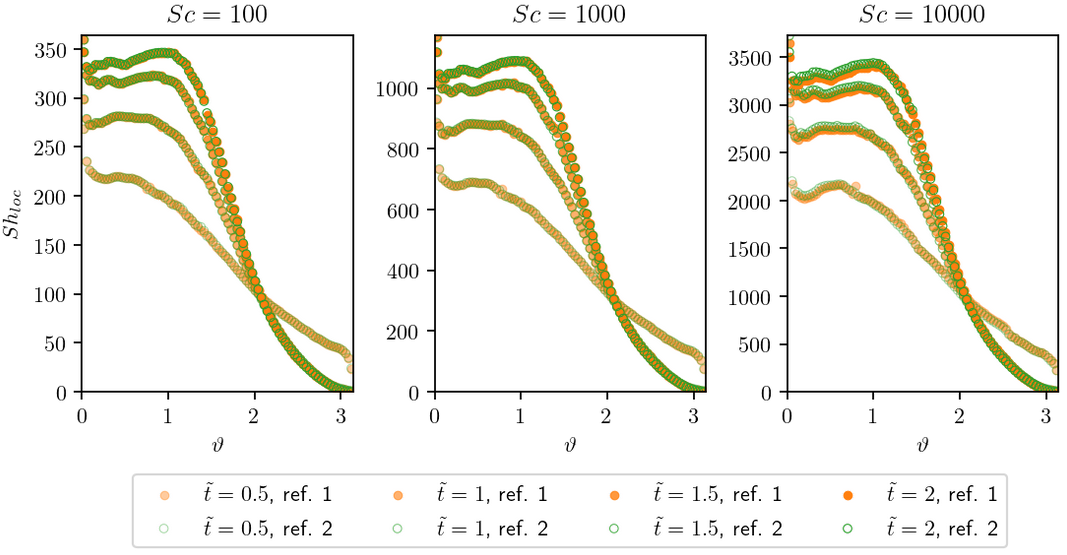Local Sherwood number for selected time instances.

Inlet boundary condition - first model:

$$\mathbf{u}_{in} = -\tilde{U}_b\mathbf{e}_y$$

$\tilde{U}_b$ - the bubble's terminal velocity

What would be a more suitable Ansatz for $\tilde{U}_b$?

1. $\tilde{U}_b = f_\theta(\tilde{t})$
2. $\tilde{U}_b = f_\theta(\tilde{t})\tilde{t}$

$\tilde{t}$ - dimensionless time; $f_\theta(\tilde{t})$ - neural network model.

Interface velocity boundary condition - second model:

$$\mathbf{u}_s = \tilde{u}_t\mathbf{t}$$

$$\tilde{u}_t = \tilde{u}_t(\vartheta, \tilde{t})$$

$\tilde{u}_t$ - tangential component of interface velocity vector.Sine and cosine functions with vertical lines at $0$ and $\pi$.

How can we enforce the correct symmetry in the model?

1. $\tilde{u}_t = f_\theta (\vartheta, \tilde{t})\mathrm{sin}(\vartheta)$
2. $\tilde{u}_t = f_\theta (\vartheta, \tilde{t})\mathrm{cos}(\vartheta)$
3. $\tilde{u}_t = f_\theta (\mathrm{sin}(\vartheta), \tilde{t})\mathrm{sin}(\vartheta)$
4. $\tilde{u}_t = f_\theta (\mathrm{cos}(\vartheta), \tilde{t})\mathrm{sin}(\vartheta)$Comparison of model prediction and reference data.

Interface deformation - third model:

$$\Delta \mathbf{x} = (\tilde{r} - r_0)\mathbf{e}_r$$

$$\tilde{r} = \tilde{r}(\vartheta, \tilde{t}) = f_\theta (\mathrm{cos}(\vartheta), \tilde{t})$$Comparison of model prediction and reference data for the bubble radius.

Mesh motion and zoom view of concentration boundary layer for $Re=569$ and $Sc=100$.Global Sherwood number $Sh$ for two different mesh resolutions (3250 and 6500 cells/diameter). ~7h, serial, 2.4 GHz.

## Outlook

What about unsupervised and reinforcement learning?

### Extracting coherent flow structures

Modal decomp. = spatial structures + temp. behavior

Flow past a surface mounted cube at $Re=40000$.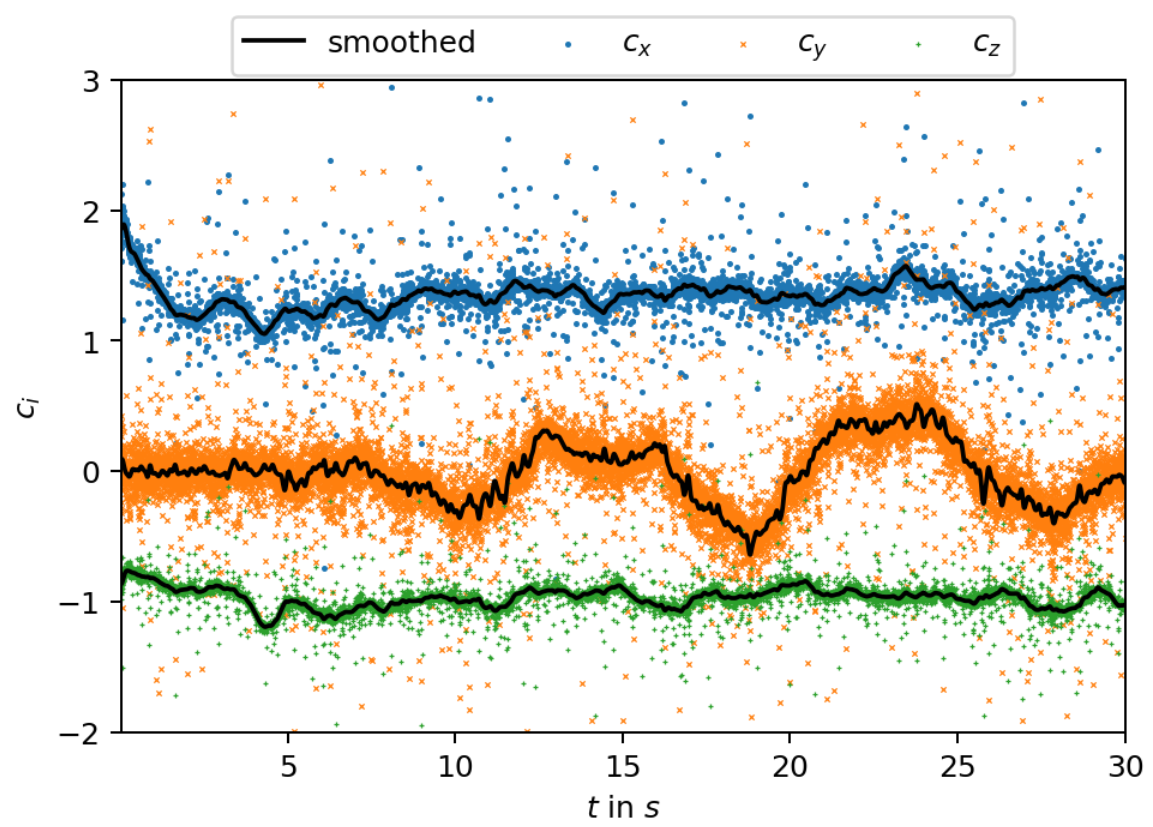Forces acting in the cube expressed as force coefficients.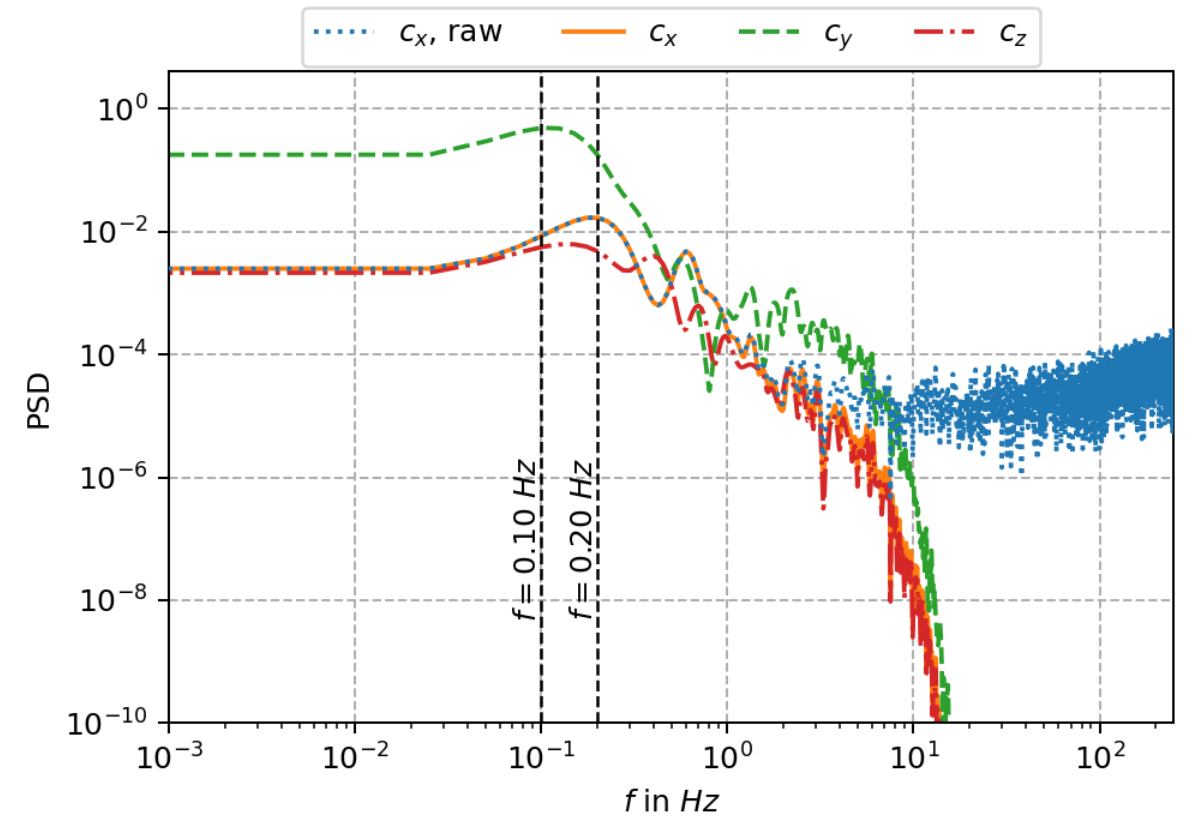Power spectral density (PSD) of force coefficients.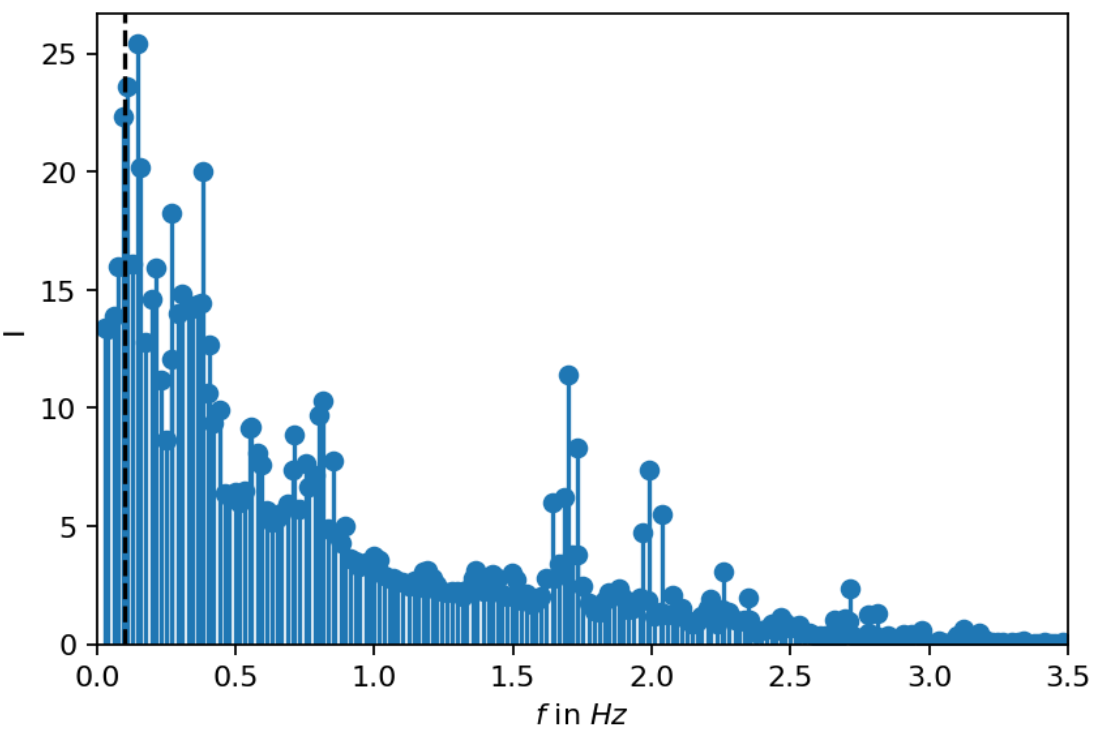Spectrum of dynamic mode decomposition (DMD).

Reconstruction of main vortex shedding mode.

Reconstruction of high-frequency vortex shedding mode.

### Closure modeling as a control problem

Closed-loop active flow control; variable inlet velocity/Reynolds number $Re(t) = 250 + 150\mathrm{sin}(\pi t)$; video by Fabian Gabriel.

LES with Smagorinsky model:

$$\nu_t = (C_s \Delta)^2 \sqrt{2\tilde{S}_{ij}\tilde{S}_{ij}}$$

Kurz et al. (2022); $C_s$ - Smagorinsky constant; $\tilde{\mathbf{S}}$ - filtered strain rate tensor.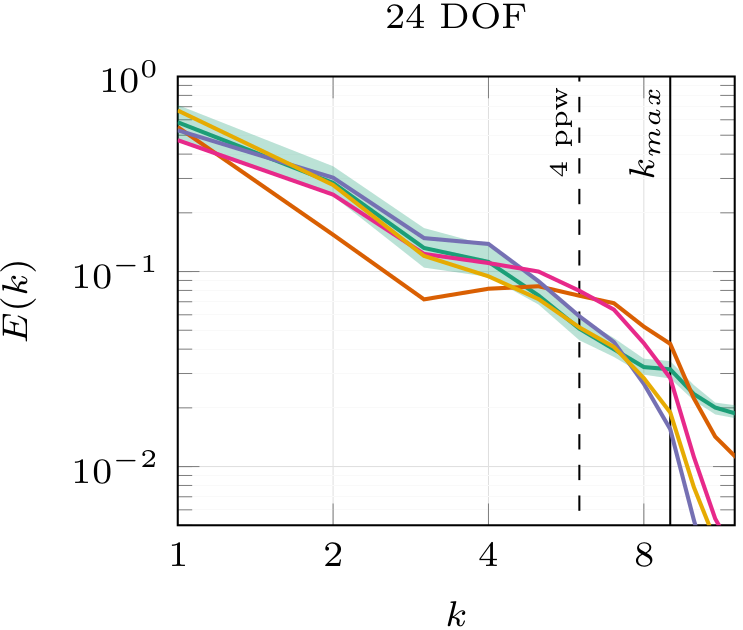Energy spectra over wave number; DNS, DRL, SSM; Kurz et al. (2022).

Where to go from here:

# THE END

### Thank you for you attention!